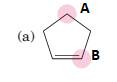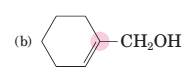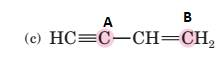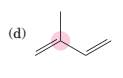# (a) Interpretation: To predict bond angles about each highlighted carbon. Concept Introduction: VSEPR predicts the geometry and the bond angles for the molecules according to the theory, the structure is predicted by the lone pairs and bond pairs present in the bond formation. Electron pair = Lone pair + Bond pair. Electron pair Hybridization Geometry Bond angles 2 sp Linear 180° 3 sp 2 Trigonal planar 120° 4 sp 3 Tetrahedra or square planar 109.5° or 90° 5 sp 3 d Trigonal bipyramidal 120° and 90° 6 sp 3 d 2 Octahedral 90°BuyFind

### Introduction to General, Organic a...

11th Edition
Frederick A. Bettelheim + 4 others
Publisher: Cengage Learning
ISBN: 9781285869759BuyFind

### Introduction to General, Organic a...

11th Edition
Frederick A. Bettelheim + 4 others
Publisher: Cengage Learning
ISBN: 9781285869759

#### Solutions

Chapter 12, Problem 12.19P
Textbook Problem

##Expert Solution
Interpretation Introduction

(a)

Interpretation:

To predict bond angles about each highlighted carbon.Concept Introduction:

VSEPR predicts the geometry and the bond angles for the molecules according to the theory, the structure is predicted by the lone pairs and bond pairs present in the bond formation.

Electron pair = Lone pair + Bond pair.

 Electron pair Hybridization Geometry Bond angles 2 sp Linear 180° 3 sp2 Trigonal planar 120° 4 sp3 Tetrahedra or square planar 109.5° or 90° 5 sp3 d Trigonal bipyramidal 120° and 90° 6 sp3 d2 Octahedral 90°

### Explanation of Solution

For Carbon A.

Number of bond pairs formed by 1 carbon=4.

Number of lone pairs present = 0.

Electron pairs =4.

Hence, the carbon A is sp3 hybridized, and the bond angle is 109...

Expert Solution
Interpretation Introduction

(b)

Interpretation:

To predict bond angles about each highlighted carbon.Concept Introduction:

VSEPR predicts the geometry and the bond angles for the molecules according to the theory, the structure is predicted by the lone pairs and bond pairs present in the bond formation.

Electron pair = Lone pair + Bond pair.

 Electron pair Hybridization Geometry Bond angles 2 sp Linear 180° 3 sp2 Trigonal planar 120° 4 sp3 Tetrahedra or square planar 109.5° or 90° 5 sp3 d Trigonal bipyramidal 120° and 90° 6 sp3 d2 Octahedral 90°
Expert Solution
Interpretation Introduction

(c)

Interpretation:

To predict bond angles about each highlighted carbon.Concept Introduction:

VSEPR predicts the geometry and the bond angles for the molecules according to the theory, the structure is predicted by the lone pairs and bond pairs present in the bond formation.

Electron pair = Lone pair + Bond pair.

 Electron pair Hybridization Geometry Bond angles. 2 sp Linear 180° 3 sp2 Trigonal planar 120° 4 sp3 Tetrahedra or square planar 109.5° or 90° 5 sp3 d Trigonal bipyramidal 120° and 90° 6 sp3 d2 Octahedral 90°
Expert Solution
Interpretation Introduction

(d)

Interpretation:

To predict bond angles about each highlighted carbon.Concept Introduction:

VSEPR predicts the geometry and the bond angles for the molecules according to the theory, the structure is predicted by the lone pairs and bond pairs present in the bond formation.

Electron pair = Lone pair + Bond pair.

 Electron pair Hybridization Geometry Bond angles. 2 sp Linear 180° 3 sp2 Trigonal planar 120° 4 sp3 Tetrahedra or square planar 109.5° or 90° 5 sp3 d Trigonal bipyramidal 120° and 90° 6 sp3 d2 Octahedral 90°

## Expert Solution

### Want to see the full answer?

Check out a sample textbook solution.

### Want to see this answer and more?

Experts are waiting 24/7 to provide step-by-step solutions in as fast as 30 minutes!*

*Response times may vary by subject and question complexity. Median response time is 34 minutes for paid subscribers and may be longer for promotional offers.© 2021 bartleby. All Rights Reserved.